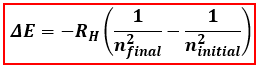# Problem: Calculate the energy, in joules, required to excite a hydrogen atom by causing an electronic transition from the n = 1 to the n = 4 principal energy level.a. 2.07 x 10-29 J b. 2.19 x 105 J c. 2.04 x 10-18 J d. 3.27 x 10-17 J e. 2.25 x 10-18 J

🤓 Based on our data, we think this question is relevant for Professor Lapeyrouse's class at UCF.

###### FREE Expert Solution

To calculate the energy required for the electronic transition, we will use the Bohr Equation shown below which relates electronic transition to the energy:ΔE = energy related to the transition
RH = Rydberg constant

ni = initial principal energy level
nf = final principal energy level

Given values:###### Problem Details

Calculate the energy, in joules, required to excite a hydrogen atom by causing an electronic transition from the n = 1 to the n = 4 principal energy level.

a. 2.07 x 10-29

b. 2.19 x 105

c. 2.04 x 10-18

d. 3.27 x 10-17

e. 2.25 x 10-18 J

What scientific concept do you need to know in order to solve this problem?

Our tutors have indicated that to solve this problem you will need to apply the Bohr and Balmer Equations concept. If you need more Bohr and Balmer Equations practice, you can also practice Bohr and Balmer Equations practice problems.

What is the difficulty of this problem?

Our tutors rated the difficulty ofCalculate the energy, in joules, required to excite a hydrog...as medium difficulty.

How long does this problem take to solve?

Our expert Chemistry tutor, Sabrina took 3 minutes and 19 seconds to solve this problem. You can follow their steps in the video explanation above.

What professor is this problem relevant for?

Based on our data, we think this problem is relevant for Professor Lapeyrouse's class at UCF.